# Sample Data: England Megalithic Monuments

Locations of megalithic monuments in England

## Details

Locations of megalithic monuments in England annotated by their names.

## Examples

### Basic Examples (1)

 In:=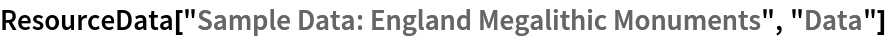Out=Summary of the spatial point data:

 In:=Out=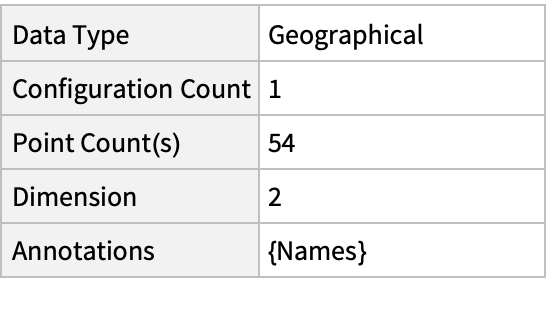### Visualizations (1)

Plot the spatial point data:

 In:=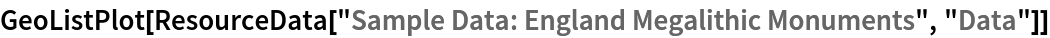Out=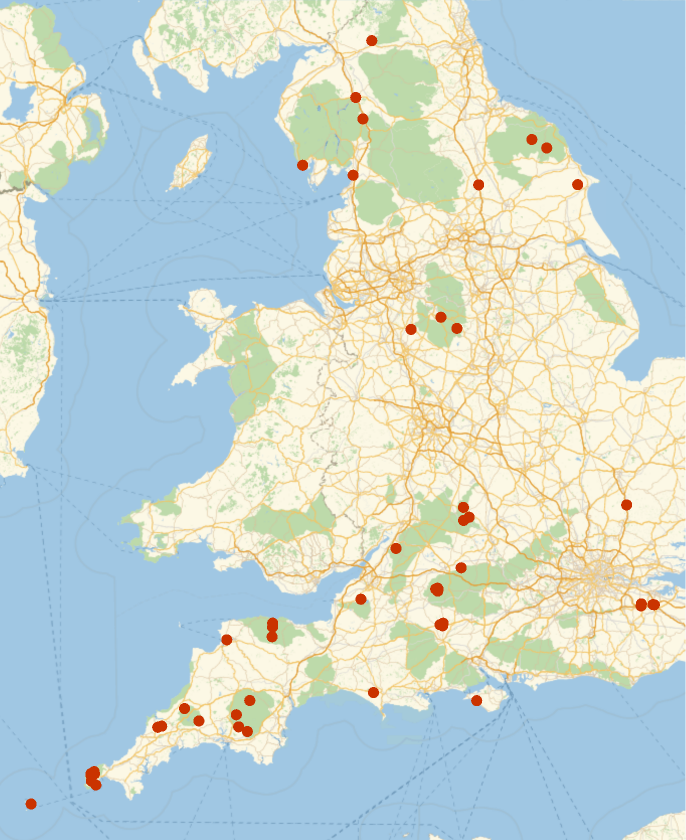### Analysis (2)

Compute probability of finding a point within given radius of an existing point - NearestNeighborG is the CDF of the nearest neighbor distribution:

 In:=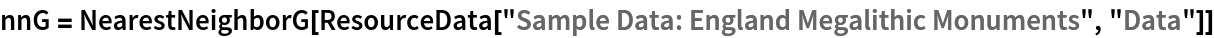Out=In:=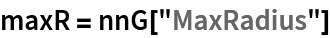Out=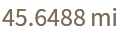In:=Out=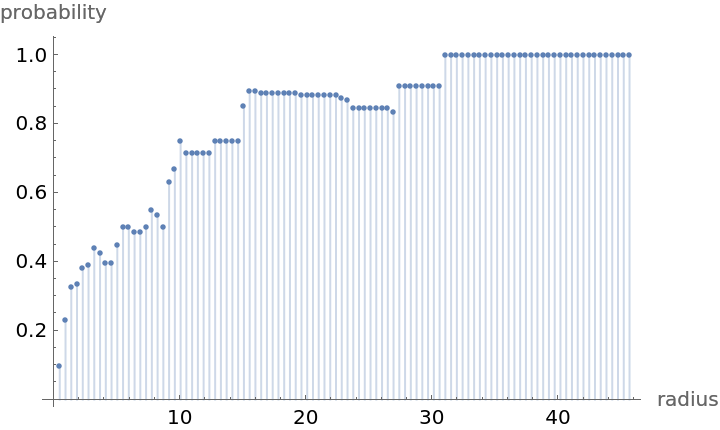Mean distance between a typical point and its nearest neighbor (for positive support distribution can be approximated via a Riemann sum of 1-CDF):

 In:=In:=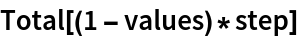Out=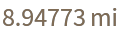Gosia Konwerska, "Sample Data: England Megalithic Monuments" from the Wolfram Data Repository (2021)